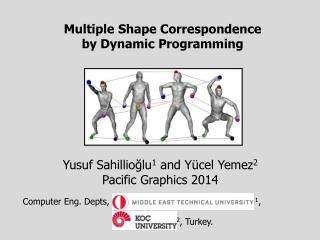DownloadDownload PresentationMultiple Shape Correspondence by Dynamic Programming

# Multiple Shape Correspondence by Dynamic Programming

Télécharger la présentation## Multiple Shape Correspondence by Dynamic Programming

- - - - - - - - - - - - - - - - - - - - - - - - - - - E N D - - - - - - - - - - - - - - - - - - - - - - - - - - -
##### Presentation Transcript

1. Multiple Shape Correspondence by Dynamic Programming Yusuf Sahillioğlu1 and Yücel Yemez2 Pacific Graphics 2014 Computer Eng. Depts, 1, 2, Turkey.

2. Problem Statement Goal: Find correspondences between all shapes in the collection: .. Key: Minimize the overall distortion. Naïve algorithm: Pick the min-distortion map between each consecutive pair . . Problem: No guarantee on the overall distortion, i.e., distortions of the maps between non-consecutive pairs, e.g., and , may be very high. Our dynamic programming algorithm addresses this multiple shape correspondence problem.

3. Applications • Improved pairwise correspondences to better perform • Shape interpolation: • Attribute transfer: • Shape registration: • Shape matching: • Statistical shape analysis:

4. Contributions • Improvedpairwise correspondences • suggests • with a total distortion sum of with a total distortion sum of • .061 + .063 + .069 = .193 .061 + .065 + .060 = .186 • .186 < .196  VS.

5. Contributions • An algorithm which explicitly minimizes the overall • distortion while enforcing consistency*. • Dynamic programming. • Novel graph structure. • No initial set of maps required. • No embedding ambiguities and errors. • Close approximation to the optimal solution. • Much faster than the alternatives. • * A set of maps is consistent iff all cycles • of maps yield the identity map.

6. Scope • A collection of initially unmatched • isometric: • or nearly isometric shapes: with arbitrary topology and scaling, as well as potential noise and non-uniform triangulation.

7. Isometry • Our method is purely isometric. • Intrinsic global property. • Similar shapes have similar metric structures. • Metric: Geodesic distance g. • Isometric distortion of a map between shapes and • A variant of the isometric distortion measures used in • Bronstein et al., SISC, 2006. • Huang et al., SGP, 2008. • Sahillioğlu and Yemez, {PAMI, 2012}, {CGF, 2013}. g g g g g g g g average for

8. Graph Representation • Same graph used in allmultiple shape corresponders so far. • Vertices: all shapes. • Edges: weighted by the matching cost of the initially • assumed pairwise maps. • General theme: Replace potentially “bad” maps with compositions of “better” maps. • Charpiat, NORDIA’09: Replaces a pairwise map b/w each shape pair by the • composition of maps on the shortest path between the two shapes. • Nguyen et al., SGP’11: Force all 3-cycles of maps return to identity. • Huang et al., SIGGRAPH Asia’12: Cycle consistency plus neighbor-preservation. • Huang and Guibas, SGP’13: Cycle consistency as the solution to a semidef. program.

9. Graph Representation • We introduce a novel graph. • Vertices: maps between shape extremities. • Edges: no weighting (no initial maps required). • Optimal path finding on this graph to solve our problem. • Accumulate & Use distortion info on growing paths via DP.

10. Algorithm All shapes in the collection:

11. Enumerate maps between consecutive shapes as graph vertices: for

12. Create paths from to and store their overall isometric distortions . m=4 m=1 m=2 m=3 m=M-1 Initially (m=1) a path is just a vertex and its distortion is easy to compute: . 3rd shape in process. Create paths ending at x-marked vertex. Compute each path distortion via dynamic programming: Eq. 6 4th shape in process. Note that existing paths may change, e.g., subpath to top node in is updated. Last shape in process. Do the same for the next x-marked graph vertex. And the same for another x-marked vertex. 5th shape in process. Save only the highlighted path with min-distortion. Output the path with min-distortion: That is, the vertices/maps on this red path.

13. Results Huang et al., SIGGRAPH Asia, 2012. Our method.

14. Future Work • Different map enumarations, e.g., to produce different types • of correspondences. • Partially isometric. • Non-isometric. • Dense (currently we match 300- samples on a high-reso • and interpolate to full dense map). • New ways of combining maps other than composition.

15. Conclusion • Rather than improving a given set of initial pairwise maps, • explicitly minimize the overall isometric distortion function • Isometric distortion minimized in the original 3D Euclidean • space wherein isometry is defined, i.e., no embedding. • Output: consistent bijections over all pairs of isometric sets. • Closely approximates the optimal solution in a fast way. • Robust to non-uniform triangulation and noise. • No restriction on topology.

16. People Yusuf, Asst. Prof. Assoc. Prof. Yücel Yemez, PhD supervisor